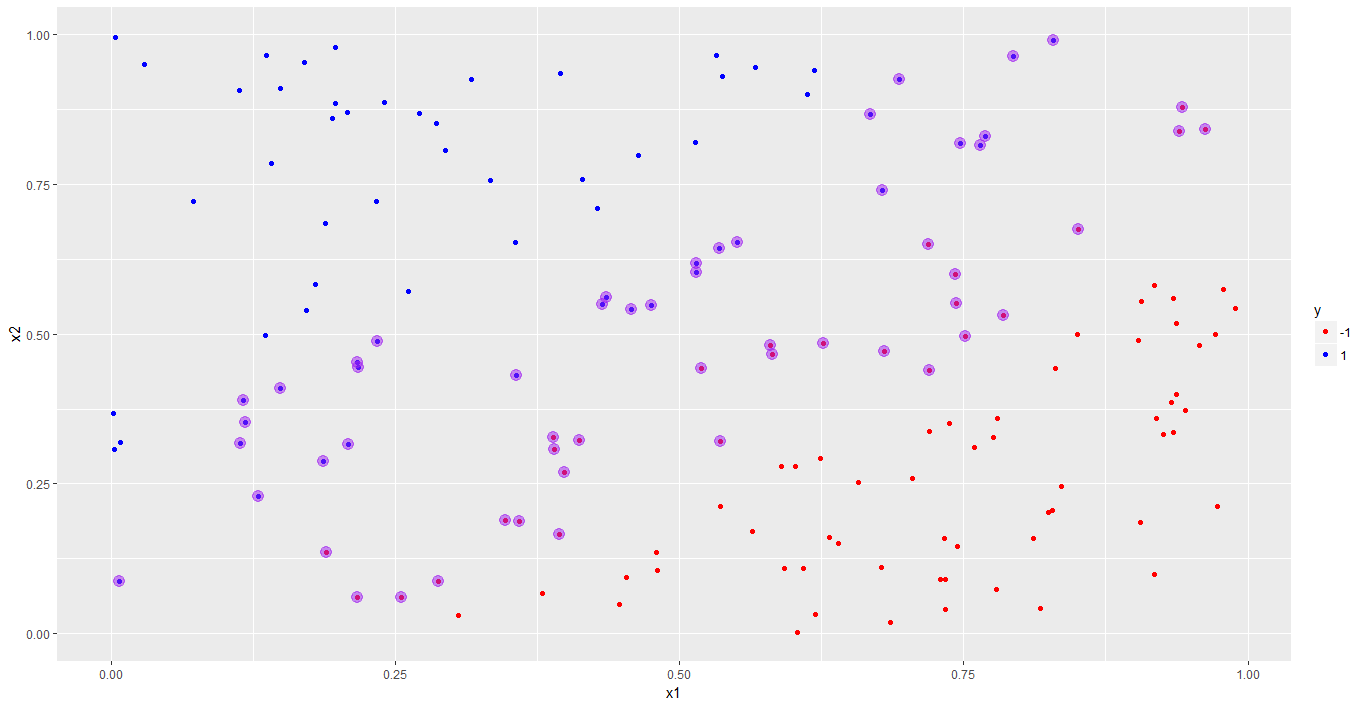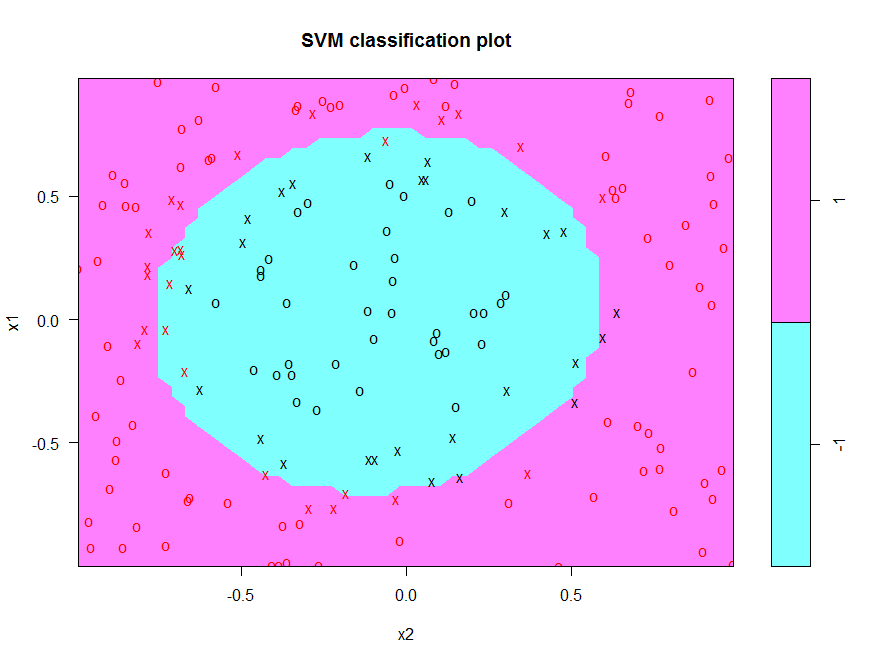Official Blog
r programming
+1

# New Course: Support Vector Machines in R

Learn about our new R course. This course will introduce a powerful classifier, the support vector machine (SVM) using an intuitive, visual approach.## Course Description

Support Vector Machines in R will help students develop an understanding of the SVM model as a classifier and gain practical experience using R’s libsvm implementation from the e1071 package. Along the way, students will gain an intuitive understanding of important concepts, such as hard and soft margins, the kernel trick, different types of kernels, and how to tune SVM parameters. Get ready to classify data with this impressive model.

## Chapter 1: IntroductionThis chapter introduces some key concepts of support vector machines through a simple 1-dimensional example. Students are also walked through the creation of a linearly separable dataset that is used in the subsequent chapter.

## Chapter 2: Support Vector Classifiers - Linear KernelsChapter 2 introduces students to the basic concepts of support vector machines by applying the svm algorithm to a dataset that is linearly separable. Key concepts are illustrated through ggplot visualizations that are built from the outputs of the algorithm and the role of the cost parameter is highlighted via a simple example. The chapter closes with a section on how the algorithm deals with multi-class problems.

## Chapter 3: Polynomial KernelsThis chapter provides an introduction to polynomial kernels via a dataset that is radially separable (i.e. has a circular decision boundary). After demonstrating the inadequacy of linear kernels for this dataset, students will see how a simple transformation renders the problem linearly separable thus motivating an intuitive discussion of the kernel trick. Students will then apply the polynomial kernel to the dataset and tune the resulting classifier.

## Chapter 4: Radial Basis Function KernelsChapter 4 builds on the previous three chapters by introducing the highly flexible Radial Basis Function (RBF) kernel. Students will create a "complex" dataset that shows up the limitations of polynomial kernels. Then, following an intuitive motivation for the RBF kernel, students see how it addresses the shortcomings of the other kernels discussed in this course.

The course requires the following prerequisites:

Introduction to R

Introduction to Machine Learning